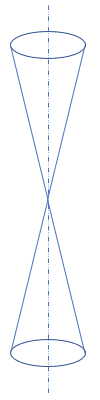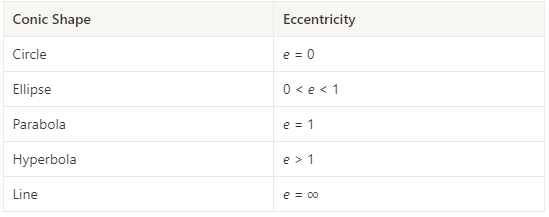## Zero Eccentricity

Which conic section has an eccentricity equal to zero?Hint
The eccentricity, $$e$$ , of a conic section indicates how close its shape is a circle.
Hint 2
The eccentricity of a parabola is one.
A conic section is a curve obtained from the intersection of a cone’s surface and a flat plane. The eccentricity, $$e$$ , of a conic section indicates how close its shape is a circle. As eccentricity grows larger, the less the shape resembles a circle. Thus,Circle